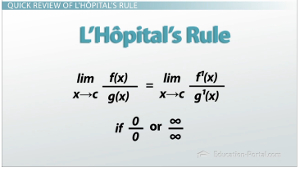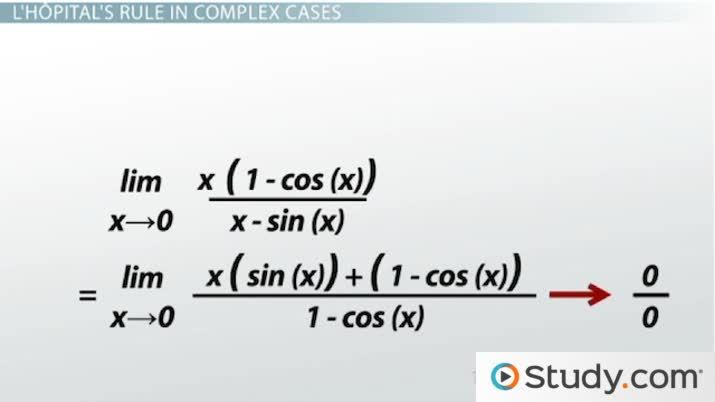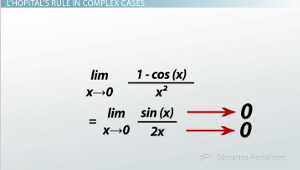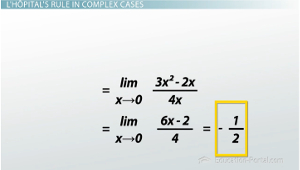# Applying L'Hopital's Rule in Complex Cases

Lesson Transcript
Instructor: Zach Pino
L'Hospital's Rule is used to find limits but also can be used to solve complex problems, such as when the limit reaches zero or infinity. Explore how applying L'Hospital's Rule in complex cases can provide a result that is easier to understand. Updated: 08/09/2021

## Quick Review of L'Hôpital's RuleL'Hôpital's rule says that if you're trying to find a limit and you end up with something like 0/0 or infinity/infinity, you can try looking at the derivatives to calculate the limit. That is, the limit as x goes to C of f(x)/g(x). If that gives you 0/0 or infinity/infinity, you can say that the limit that you were originally looking for is equal to the limit as x goes to C of f'(x)/g'(x).An error occurred trying to load this video.

Try refreshing the page, or contact customer support.

Coming up next: Linearization of Functions

### You're on a roll. Keep up the good work!

Replay
Your next lesson will play in 10 seconds
• 0:06 Quick Review of…
• 0:35 Example of L'H?pital's…
• 2:50 Second Example
• 4:19 Third Example
• 7:39 Lesson Summary
Save Save

Want to watch this again later?

Timeline
Autoplay
Autoplay
Speed Speed

## Example of L'Hôpital's Rule in Complex Cases

Sometimes though, L'Hôpital's rule doesn't quite give you what you're looking for. Like in the case of the limit as x goes to zero of (1 - cos(x)) / x^2. Now at x=0, I have zero on the top and zero on the bottom, so this tells me that I should use L'Hôpital's rule. I do this, and I find that the limit as x goes to zero of (1 - cos(x)) / x^2 is the same as the limit as x goes to zero of sin(x) / 2x, because sin(x) is the derivative of 1 - cos(x), and 2x is the derivative of x^2. Here's the problem though; the limit as x goes to zero of sin(x) is 0, and the limit as x goes to zero of 2x is 0. Hmm, well, this is a problem. We use L'Hôpital's rule to avoid this in the first place. Now what?Well, sin(x) / 2x is really just another function, right? So we can use L'Hôpital's rule on this. Now my new f(x) is sin(x), and my new g(x) is 2x. If I use that in L'Hôpital's rule, then I say the limit as x goes to zero of sin(x) / 2x is equal to the limit as x goes to zero of cos(x) - which is the derivative of sin(x) - divided by 2, which is the derivative of 2x. Finally, as x goes to zero, cos(x) goes to 1, and as x goes to zero, 2 stays 2. So I finally have a limit that's not 0/0. What I end up with is the limit as x goes to zero of (1 - cos(x)) / x^2 is equal to 1/2, and I found this just by repeatedly applying L'Hôpital's rule.

## Second Example

So we see this kind of thing a lot when we're looking at things like trigonometric functions and polynomials. Like the example limit as x goes to zero of (x^3 - x^2) / (2x^2). Now at x=0, both the top and the bottom are zero, so this limit is 0/0. I apply L'Hôpital's rule using the top - f(x)=x^3 - x^2 - and the bottom, g(x), being 2x^2. I find the derivatives of those, and I plug them in. I end up with the limit as x goes to zero of (3x^2 - 2x) / 4x. Well, once again this limit is giving me 0/0, so I'm going to apply L'Hôpital's rule again. My new f(x) is 3x^2 - 2x from the top here, and my new g(x) is 4x. I find the derivatives, I plug them in, and I get the limit as x goes to zero of (6x - 2)/4. Well that limit exists, and that limit is -2/4, or -1/2. So from all this, these repeated applications of L'Hôpital's rule, I find that the limit as x goes to zero of (x^3 - x^2) / (2x^2) is -1/2.To unlock this lesson you must be a Study.com Member.

### Register to view this lesson

Are you a student or a teacher?